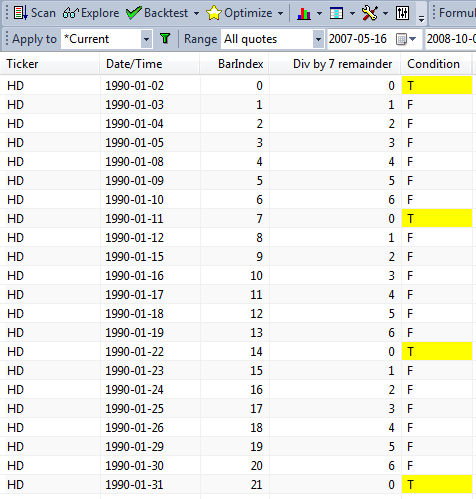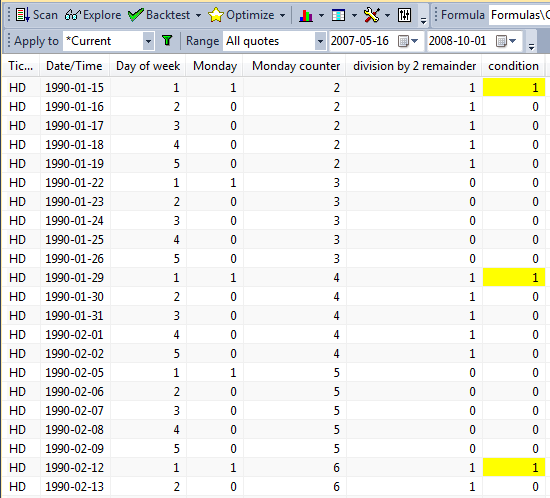#amibroker

### Detecting N-th occurrence of a condition using modulus operator

Modulus (%) is an operator that returns the reminder from integer division. It is very helpful to create counters that wrap-around at user-specified N.

In order to define a condition, which returns True every Nth bar, the easiest way is just to use % (modulus) operator. If we apply modulus to consecutive numbers such as BarIndex() – then calculating the reminder from integer division of barindex by N will return 0 every Nth bar (on bars that are divisible by N). We can use the following exploration to demonstrate that:

`n = 7;bi = BarIndex();condition = bi % n == 0;Filter = 1;AddColumn( bi, "BarIndex", 1 );AddColumn( bi % n , "Div by 7 remainder ", 1 );AddColumn( IIF( condition, 'T', 'F' ), "Condition",           formatChar, colorDefault, IIf( condition, colorYellow, colorDefault ) )`Since the remainder from division by 7 will equal zero only for the multiples of 7, then we will have our condition True every 7th bar (as marked in the above exploration results with T letter on yellow background).

Using the same technique we can also count occurrences of certain criteria and then apply the % operator. For example – let us say we want to test a rotational strategy, where we rotate our portfolio every 2nd Monday. To detect such condition in our code we need to first identify all Monday bars, then count them and use % operator to divide such count by 2.

The following exploration shows the calculations of the condition we look for:

`Filter = 1;AddColumn( DayOfWeek(), "Day of week", 1 );AddColumn( Mon, "Monday", 1 );AddColumn( countMon, "Monday counter", 1 );AddColumn( countMon % 2 == 0, "division by 2 remainder", 1 );AddColumn( rotation, "condition", 1, colorDefault, IIf( rotation, colorYellow, colorDefault) )`Here is the formula showing how to code these technique for the rotational back-test:

`SetBacktestMode( backtestRotational );posScore = 100 - RSI(); // sample scoring indicatorSetPositionSize( 10, spsShares ); // sample position sizingMon = DayOfWeek() == 1; // identify MondayscountMon = Cum( Mon ); // count Mondays// rotate only on Monday, every 2nd onerotation = Mon AND (countMon % 2 == 0 );PositionScore = IIf( rotation, posScore, scoreNoRotate )`